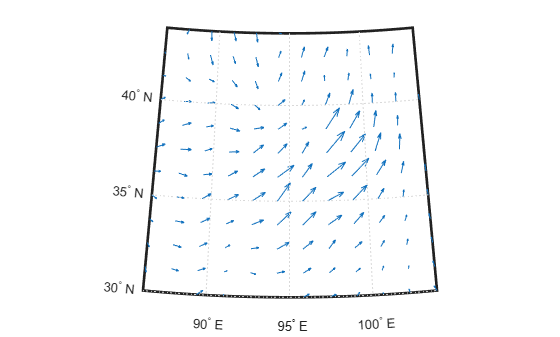# quiverm

Project 2-D quiver plot on map axes

## Syntax

```h = quiverm(lat,lon,deltalat,deltalon) h = quiverm(lat,lon,deltalat,deltalon,LineSpec) h = quiverm(lat,lon,deltalat,deltalon,LineSpec,'filled') h = quiverm(lat,lon,deltalat,deltalon,scale) h = quiverm(lat,lon,deltalat,deltalon,LineSpec,scale,'filled') ```

## Description

`h = quiverm(lat,lon,deltalat,deltalon)` displays velocity vectors with components `(deltalat,deltalon)` at the geographic points `(lat,lon)` on displayed map axes. All four inputs should be in the `AngleUnits` of the map axes. The inputs `deltalat` and `deltalon` determine the direction of the vectors in latitude and longitude, respectively. The function automatically determines the length of these vectors to make them as long as possible without overlap. The object handles of the displayed vectors can be returned in `h`.

`h = quiverm(lat,lon,deltalat,deltalon,LineSpec)` specifies the line style, marker, and color. If you use markers, they are plotted at the start points of the vectors, i.e., the input points (`lat`,`lon`).

`h = quiverm(lat,lon,deltalat,deltalon,LineSpec,'filled')` results in the filling in of any symbols specified by `LineSpec`.

`h = quiverm(lat,lon,deltalat,deltalon,scale)` and ```h = quiverm(lat,lon,deltalat,deltalon,LineSpec,scale,'filled')``` alter the automatically calculated vector lengths by multiplying them by the scalar value `scale`. For example, if `scale` is 2, the displayed vectors are twice as long as they would be if `scale` were 1 (the default). When scale is set to 0, the automatic scaling is suppressed, and the length of the vectors is determined by the inputs. In this case, the vectors are plotted from (`lat`,`lon`) to (`lat+deltalat`,`lon+deltalon`).

## Examples

collapse all

`load coastlines`

Setup an axes specifying latitude and longitude limits. Plot the coast line data.

```axesm('eqaconic','MapLatLimit',[30 60],'MapLonLimit',[-10 10]) framem; plotm(coastlat,coastlon)```Plot quiver vectors in a direction corresponding to +5 degrees latitude and +3 degrees longitude. Use automatic scaling.

```lat0 = [50 39.7]; lon0 = [-5.4 2.9]; deltalat = [5 5]; deltalon = [3 3]; quiverm(lat0,lon0,deltalat,deltalon,'r')```collapse all

### Line Style, Marker, and Color

Specify the line style, marker, and color as a character vector or string containing symbols. The symbols can appear in any order. You do not need to specify all three characteristics (line style, marker, and color). For example, specify a red dashed line using `'--r'`.

Line StyleDescriptionResulting Line
`'-'`Solid line`'--'`Dashed line`':'`Dotted line`'-.'`Dash-dotted lineMarkerDescriptionResulting Marker
`'o'`Circle`'+'`Plus sign`'*'`Asterisk`'.'`Point`'x'`Cross`'_'`Horizontal line`'|'`Vertical line`'s'`Square`'d'`Diamond`'^'`Upward-pointing triangle`'v'`Downward-pointing triangle`'>'`Right-pointing triangle`'<'`Left-pointing triangle`'p'`Pentagram`'h'`HexagramColor NameShort NameRGB TripletAppearance
`'red'``'r'``[1 0 0]``'green'``'g'``[0 1 0]``'blue'``'b'``[0 0 1]``'cyan'` `'c'``[0 1 1]``'magenta'``'m'``[1 0 1]``'yellow'``'y'``[1 1 0]``'black'``'k'``[0 0 0]``'white'``'w'``[1 1 1]`